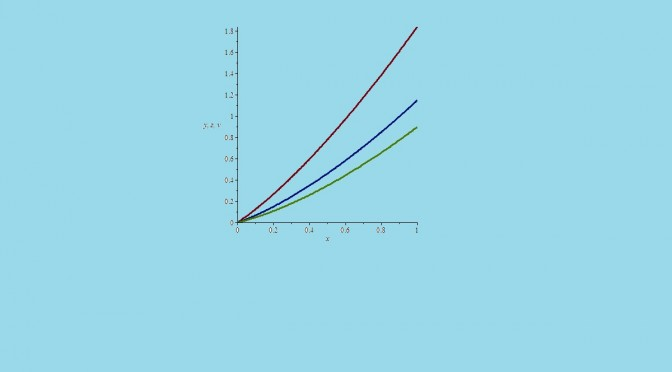A continuous differential equation with no solution

Most of Cauchy existence theorems for a differential equation
\begin{equation}
\textbf{x}^\prime = \textbf{f}(t,\textbf{x})
\end{equation} where $$t$$ is a real variable and $$\textbf{x}$$ a vector on a real vectorial space $$E$$ are valid when $$E$$ is of finite dimension or a Banach space. This is however not true for the Peano existence theorem.

Take for $$E$$ the space of real sequences converging to $$0$$ for the norm $$\Vert \textbf{x} \Vert = \sup_n \vert x_n \vert$$. For all $$\textbf{x} \in E$$ let’s note $$\textbf{y}=\textbf{f}(\textbf{x})$$ the sequence $$(y_n)$$ defined by:
\begin{equation*}
y_n=\sqrt{\vert x_n \vert} + \frac{1}{n+1}
\end{equation*} As $$\lim\limits_{n \to +\infty} y_n=0$$, $$\textbf{y}$$ belongs to $$E$$. As the real function $$\sqrt{\vert x \vert}$$ is uniformly continuous on $$\mathbb{R}$$, the function $$\textbf{y}=\textbf{f}(\textbf{x})$$ is continuous on $$E$$.

However, we’ll prove that the differential equation
\begin{equation}
\textbf{x}^\prime = \textbf{f}(\textbf{x})
\label{eq:eqglobal}
\end{equation} has no solution in $$E$$ with initial condition $$\textbf{x}(0)=\textbf{0}$$. If $$\textbf{u}$$ was a solution, there would exist a sequence $$\textbf{u}(t)=[u_n(t)]$$ of differentiable functions in a neighborhood of $$0$$ satisfying:
\begin{equation}
u_n^\prime(t)=\sqrt{\vert u_n(t) \vert} + \frac{1}{n+1}
\label{eq:eqwithn}
\end{equation} and $$u_n(0)=0$$ for all $$n \in \mathbb{N}$$.

By direct integration it can be proved that each equation \eqref{eq:eqwithn} has a unique solution satisfying $$u_n(0)=0$$. For all $$n \in \mathbb{N}$$, $$u_n$$ is an odd function defined on all $$\mathbb{R}$$ and verifying for $$t \ge 0$$: $$u_n(t) \ge \frac{t^2}{4}$$. This last inequality, valid for all $$n \in \mathbb{N}$$ contradicts the ability for $$\textbf{u}$$ to belongs to $$E$$. Therefore, the equation \eqref{eq:eqglobal} has no solution.

This counterexample is from the French mathematician Jean Dieudonné.

4 thoughts on “A continuous differential equation with no solution”

1.Tadashi says:

Dear Jean-Pierre Merx,

I done my calculations again and now I see that I was mistaken. Sorry for the trouble and thanks for providing the proof, now the inequality seems clear to me. 🙂

Best regards,

2.Tadashi says:

Dear Jean-Pierre Merx, congratulations for the wonderful site!
I wish success for the site and I’m looking forward for more counterexamples 🙂

Between, I think there is a mistake in the last inequality of this counterexample, i.e. u_n(t) ≥ t ²/4 for t ≥ 0.
Consider for instance that n = 2. Then as the following plot suggests, there exists a q ≥ 0 such that the inequality is not valid for all t ≥ q.
Is there another way to prove that u doesn’t belongs to E?
Thanks a lot and best regards!

1.Jean-Pierre Merx says:

In fact the inequality $$u_n(t) \ge \frac{t^2}{4}$$ can be proven either by direction integration or by considering the inequality $$u_n^\prime(t) > \sqrt{u_n(t)}$$ valid for all $$t>0$$.